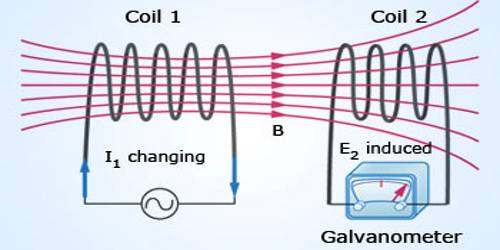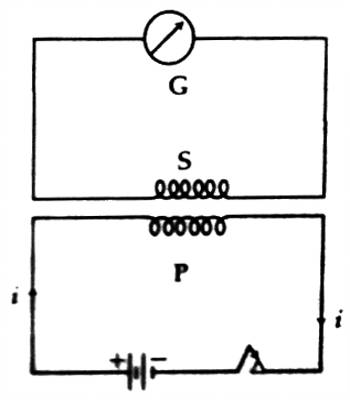# Explanation of Mutual Induction

Explanation of Mutual Induction

Electromagnetic induction which is produced in the secondary coil due to the non-uniform flow of current in the primary coil is called mutual induction. It is the production of an electromotive force in a circuit by a change in the current in an adjacent circuit which is linked to the first by the flux lines of a magnetic field.

Explanation: Let us consider two coils P and S. They are kept very close to each other. A battery and a tapping key are connected to the coil P [Figure). It is called the primary coil. A galvanometer is connected to the coil, S. It is called the secondary coil.Now, if a current is allowed to flow in the primary coil P by pressing the tapping key, then the galvanometer in the secondary coil S will give deflection. If the circuit is disconnected by releasing the key, then also the galvanometer will show deflection. But this deflection is in the opposite direction to the previous one. The reason for the flow of current in the secondary coil when the circuit in the primary coil is disconnected is described below.

If the tapping key is pressed, the current in the circuit starts increasing from zero to a fixed value. Due to this change of current the magnetic flux in the secondary coil changes: consequently, according to Faraday’s Law, induced electromotive force is produced in the secondary coil. As a result, current flows in the secondary coil. Due to this current, the galvanometer shows deflection. Now, if the tapping key is released, then the circuit becomes disconnected and current in the circuit falls from the constant value to zero. During this time magnetic flux in the secondary coil changes which creates electromotive force. The direction of this electromotive force is opposite to the previous one, so, also the direction of the current. As a result, the galvanometer gives deflection in the opposite direction.